# Decomposition¶

Examples concerning the sklearn.decomposition module.Beta-divergence loss functions

Beta-divergence loss functions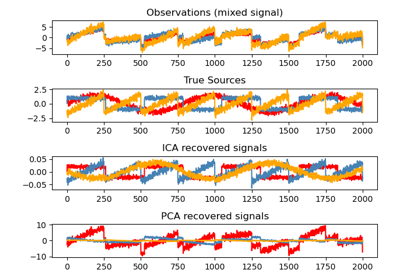Blind source separation using FastICA

Blind source separation using FastICA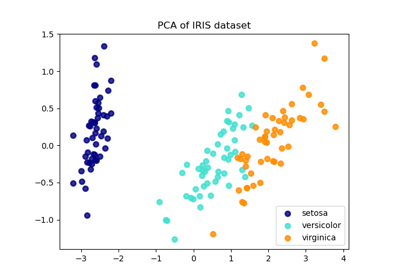Comparison of LDA and PCA 2D projection of Iris dataset

Comparison of LDA and PCA 2D projection of Iris dataset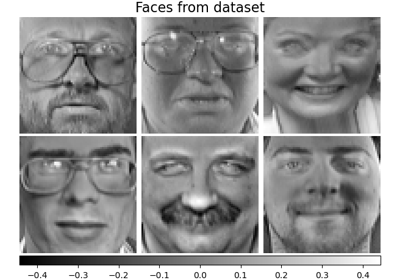Faces dataset decompositions

Faces dataset decompositions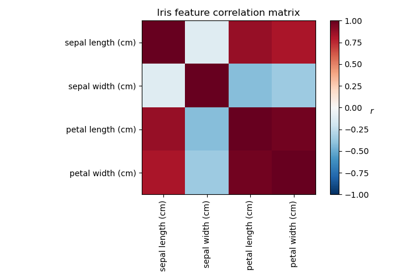Factor Analysis (with rotation) to visualize patterns

Factor Analysis (with rotation) to visualize patterns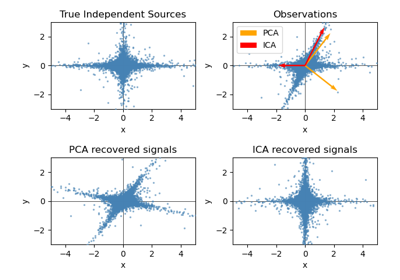FastICA on 2D point clouds

FastICA on 2D point clouds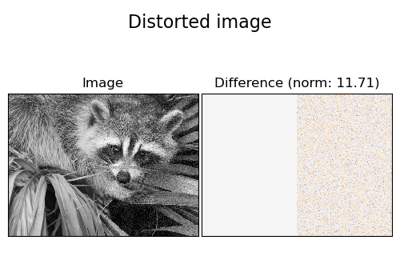Image denoising using dictionary learning

Image denoising using dictionary learning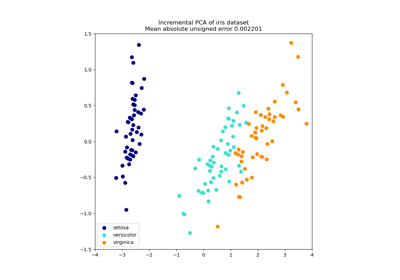Incremental PCA

Incremental PCA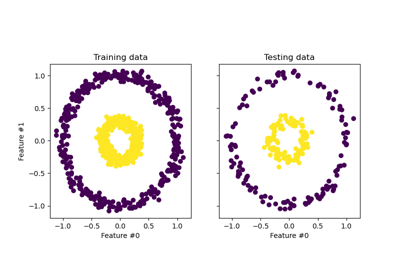Kernel PCA

Kernel PCA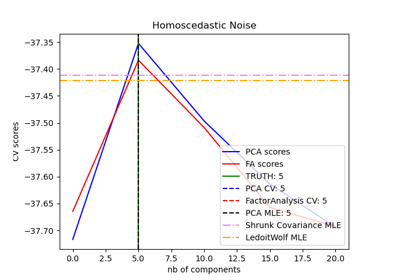Model selection with Probabilistic PCA and Factor Analysis (FA)

Model selection with Probabilistic PCA and Factor Analysis (FA)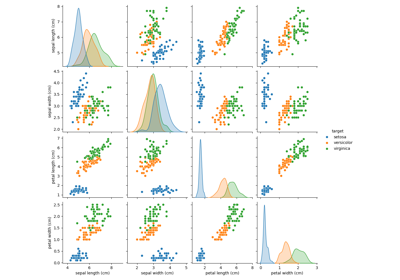PCA example with Iris Data-set

PCA example with Iris Data-setPrincipal components analysis (PCA)

Principal components analysis (PCA)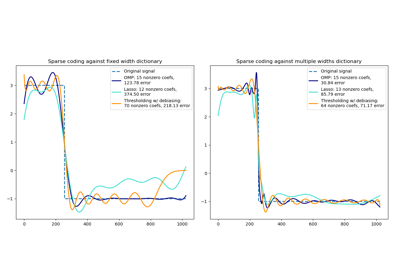Sparse coding with a precomputed dictionary

Sparse coding with a precomputed dictionary